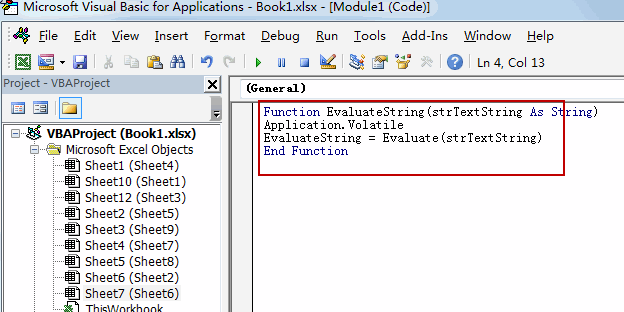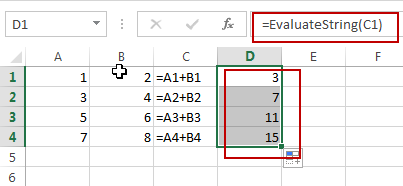# How to Convert a String into a Formula in Excel

This post will guide you how to convert a string into a formula in Excel. How do I turn test strings to a formula for calculation.

## Convert a String into a Formula

Assuming that you have a list of data in range C1:C4, which contains text string (Actually, they are all formulas, but they are shown as text string format), and you want to convert those test string into a formula for calculation. How to do it. You can create your own Excel Functions with VBA Code. It is also called as a User Defined Function. And it work the same way as Excel’s build-in standard functions. Just do the following steps:

#1 open your excel workbook and then click on “Visual Basic” command under DEVELOPER Tab, or just press “ALT+F11” shortcut.#2 then the “Visual Basic Editor” window will appear.

#3 click “Insert” ->”Module” to create a new module.#4 paste the below VBA code into the code window. Then clicking “Save” button.```Function EvaluateString(strTextString As String)
Application.Volatile
EvaluateString = Evaluate(strTextString)
End Function```

#5 back to the current worksheet, then type the following formula in a blank cell, and then press Enter key. And drag the AutoFill Handle over to other cells.

=EvaluateString(C1)You would notice that all  strings in range C1:C4 have been converted as formulas.

Related Posts

How to Extract First Letter from Each Word in a Cell in Excel

This post will guide you how to extract first letter from each word in a given cell in Excel. How do I extract the first letter of each word in a range of names in your current worksheet in Microsoft ...

How to ignore Blank Cells while Concatenating cells in Excel

This post will guide you how to concatenate cells but ignore all blank cells in your worksheet in Excel. How do I concatenate cells but ignore blank cells with a formula in Excel. How to create a concatenate formula to ...

How to Calculate the Average Excluding the Smallest & Highest Numbers in Excel

Calculating the average for a batch of data is frequently used in our daily life. But for some cases like statistic the average score in a competition, or price analysis, we often calculate the average excluding the smallest and highest ...

How to Get the Maximum or Minimum Absolute Value in Excel

It is easy to find the maximum or minimum value in a batch of data in excel, but if this batch of numbers contains both positive and negative numbers, the maximum or minimum absolute value cannot be found out by ...

How to Count Only Numbers in Bold in a Range of Cells in Excel

This post will guide you how to Count only numbers with bold style in a range of cells in Excel. How do I Count on cells with bold font within a range of cells using User defined function in Excel ...

Sidebar